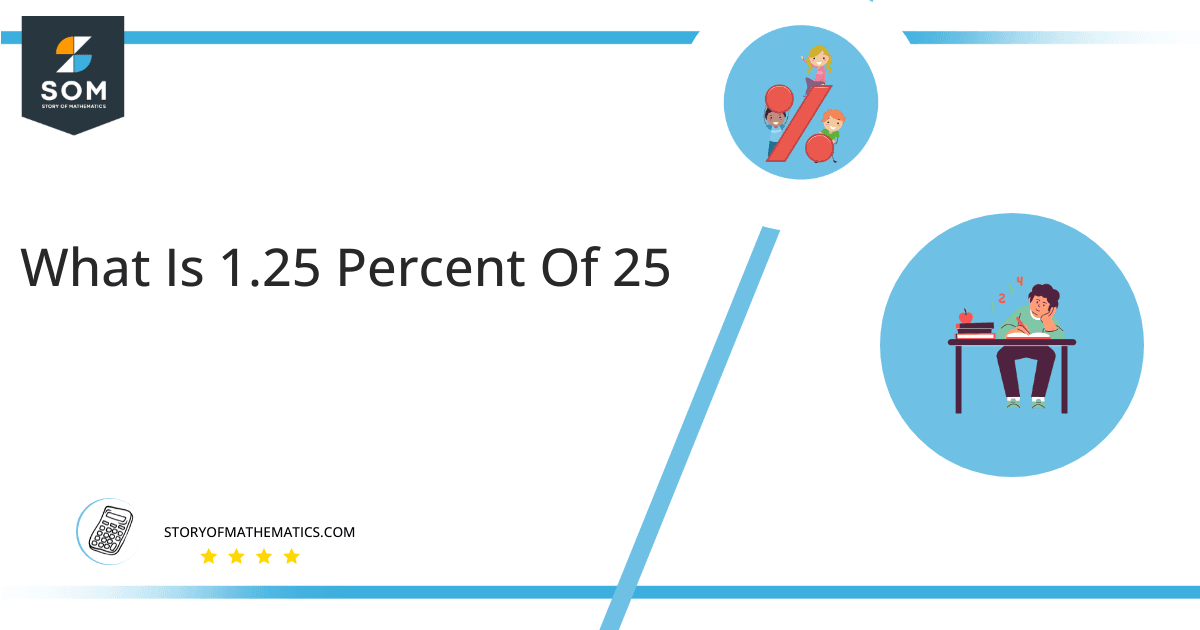# What Is 1.25 Percent of 25 + Solution with Free Steps?The 1.25 percent of 25 is equal to 0.3125. It can be easily calculated by dividing 1.25 by 100 and multiplying the answer with 25 to get 0.3125.

The easiest way to get this answer is by solving a simple mathematical problem of percentages. You need to find 1.25% of 25 for some sale or real-life problem. Divide 1.25 by 100, multiply the answer by 25, and get the 1.25% of 25 value in seconds.

This article will explain the full process of finding any percentage value from any given quantity or number with easy and simple steps.

## What is 1.25 percent of 25?

The 1.25 percent of 25 is 0.3125.

The percentage can be understood with a simple explanation. Take 25, and divide it into 100 equal parts. The 33 parts from the total of 100 parts is called 33 percent, which is 0.3125 in this example.

## How To Calculate 1.25 percent of 25?

You can find 1.25 percent of 25 by some simple mathematical steps explained below.### Step 1

Firstly, depict 1.25 percent of 25 as a fractional multiple as shown below:

1.25% x 25

### Step 2

The percentage sign % means percent, equivalent to the fraction of 1/100.

Substituting this value in the above formula:

= (1.25/100) x 25

### Step 3

Using the algebraic simplification process, we can arithmetically manipulate the above equation as follows:

= (1.25 x 25) / 100

= 31.25 / 100

= 0.3125This percentage can be represented on a pie chart for visualization. Let us suppose that the whole pie chart represents the 25 values. Now, we find 1.25 percent of 25, which is 0.3125. The area occupied by the 0.3125 value will represent the 1.25 percent of the total 25 values. The remaining region of the pie chart will represent 98.75 percent of the total 25 values. The 100% of 25 will cover the whole pie chart as 25 is the total value.

Any given number or quantity can be represented in percentages to better understand the total quantity. The percentage can be considered a quantity that divides any number into hundred equal parts for better representation of large numbers and understanding.

Percentage scaling or normalization is a very simple and convenient method of representing numbers in relative terms. Such notations find wide application in many industrial sectors where the relative proportions are used.